MORE IN Analog Electronics
Total marks: --
Total time: --
INSTRUCTIONS
(1) Assume appropriate data and state your reasons
(2) Marks are given to the right of every question
(3) Draw neat diagrams wherever necessary

1 (a) With respect to a semiconductor diode, explain the following:
i) Transition and diffusion capacitance.
ii) Reverse recovery time.
6 M
1 (b) Explain the operation of the circuit shown in Fig Q1 (b). Draw output waveform and transfer characteristic [Assume ideal diode.].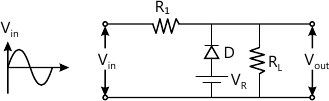7 M
1 (c) Write the procedure for analyzing the clamping circuit. Determine output voltage for the network shown in Fig. Q1(c). Assume f=1000Hz and ideal diode.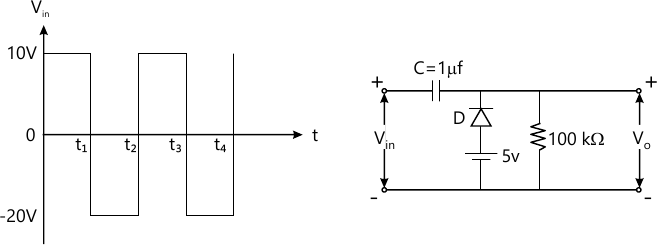7 M

2 (a) With circuit diagram, explain Emitter stabilized bias circuit. Write the necessary equation.
7 M
2 (c) For the circuit shown in Fig Q2(c), find IC, VB, VE, RI and S(ICO).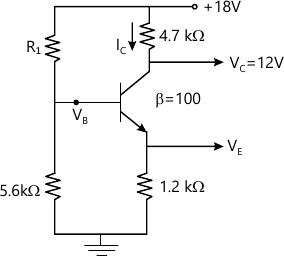7 M

3 (a) Draw the circuit diagram of common Emitter fixed bias configuration. Derive the expression for Zi, Zo, Av using re model.
8 M
3 (b) For the network shown in Fig.Q3(b), determine re, Zi, Zo, A<;sub>v and AI.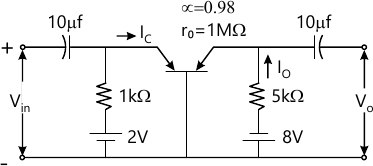6 M
3 (c) What are the advantages of h-parameters?
6 M

4 (a) Discuss the effect of various capacitors on frequency response.
4 M
4 (b) What is Miller effect? Prove that Miller effect capacitance Cmi=(1-Av) Cf and $C_{mo}- \left ( 1- \dfrac {1}{A_v} \right )C_f$
8 M
4 (c) Determine the high frequency response of the amplifier circuit shown in Fig. Q4(c). Draw the frequency response curve.

β = 100
Cbe = 20PF
Cbc = 4PF
hie = 1100
Cwi = 6 PF
Cwo = 8PF
Cce = 1PF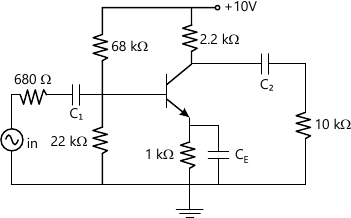8 M

5 (a) Explain the need of cascading amplifier? Draw and explain the block diagram of two-stage cascade amplifier.
4 M
5 (b) With block diagram, explain the concept of feedback amplifier. If an amplifier has mid-band voltage gain (Av mid) of 1000 with fL=50Hz and fH=50 KHz, if 5% feedback is applied then calculate fL and fH with feedback.
8 M
5 (c) Derive the expression for input resistance (Rif) for feedback amplifier employing current series feedback.
8 M

6 (a) A series fed class-A amplifier shown in Fig.Q6(a) operates from dc source and applied sinusoidal input signal generates peak base current 9 mA. Calculate ICQ, VCEQ, PDC, Pac and efficiency. Assume β=50 and VBE=0.7 V.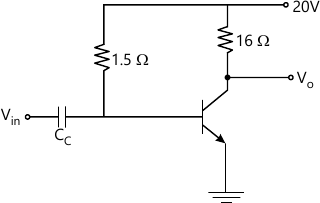6 M
6 (b) What is harmonic distortion? Explain the three point method of calculating the second harmonic distortion.
8 M
6 (c) Explain the working of complementary symmetry class-B amplifier.
6 M

7 (a) With circuit diagram, explain RC-phase shift oscillator using BJT.
8 M
7 (b) In a transistorized Hartley oscillator the two inductances are 2mH and 20μH, while the frequency is to be changed from 950 KHz to 2050 KHz. Calculate the range over which the capacitor is to be varied.
4 M
7 (c) With circuit diagram, explain the working of crystal oscillator in series resonant mode. A crystal has the following parameters L=0.334H, C=0.065 pF and R=5.5 KΩ. Calculate the resonant frequency.
8 M

8 (a) Compare FET over BJT.
6 M
8 (b) With equivalent circuit obtain the expression for Zi and Zo for JFET self bias with unbypassed R.
8 M
8 (c) The fixed bias configuration shown in Fig. Q8(c) has VGSQ=-2V, IDQ=5.625mA with IDSS=10mA, Vp=-8V and YDS=40 μs determine gm, rd, Zo and Av.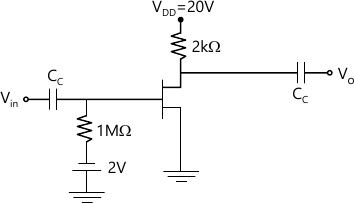6 M

More question papers from Analog Electronics